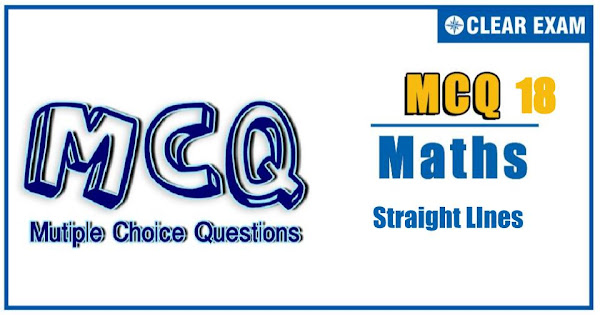## [LATEST]\$type=sticky\$show=home\$rm=0\$va=0\$count=4\$va=0

The unit Straight Line holds sheer significance in the JEE Advanced and other engineering exams. It has a weightage of 6% pairing with Circles. With focused practice good marks can be fetched from this section. These questions are important in achieving your success in JEE and Other Engineering Exams..

Q1. Statement 1: Let the vertices of a ∆ABC are A(-5,-2),B(7,6) and C(5,-4). Then coordinates of circumcentre are (1, 2)

Statement 2: In a right angle triangle, midpoint of hypotenuse is the circumcenter of the triangle
•  Statement 1 is True, Statement 2 is True; Statement 2 is correct explanation for Statement 1
•  Statement 1 is True, Statement 2 is True; Statement 2 is not correct explanation for Statement 1
•  Statement 1 is True, Statement 2 is False
•  Statement 1 is False, Statement 2 is True
Q2. Statement 1: Each point on the line y-x+12=0 is equidistant from the lines 4y+3x-12=0,3y+4x-24=0

Statement 2: The locus of a point which is equidistant from two given lines is the angular bisector of the two lines
•  Statement 1 is True, Statement 2 is True; Statement 2 is correct explanation for Statement 1
•  Statement 1 is True, Statement 2 is True; Statement 2 is not correct explanation for Statement 1
•  Statement 1 is True, Statement 2 is False
•  Statement 1 is False, Statement 2 is True
Q3. Statement 1: If (a1 x+b1 y+c1 )+(a2 x+b2 y+c2 )+(a3 x+b3 y+c3 )=0, then lines a1 x+b1 y+c1=0,a2 x+b2 y+c2=0 and a3 x+b3 y+c3=0 cannot be parallel.

Statement 2: If sum of the equations for three straight lines is identically zero, then they are either concurrent or parallel
•  Statement 1 is True, Statement 2 is True; Statement 2 is correct explanation for Statement 1
•  Statement 1 is True, Statement 2 is True; Statement 2 is not correct explanation for Statement 1
•  Statement 1 is True, Statement 2 is False
•  Statement 1 is False, Statement 2 is True

Q4. Statement 1: If -2h=a+b, then one line of the pair of lines ax2+2hxy+by2=0 bisects the angle between coordinate axes in positive quadrant

Statement 2: If ax+y(2h+a)=0 is a factor of ax2+2hxy+by2=0, then b+2h+a=0
•  Statement 1 is True, Statement 2 is True; Statement 2 is correct explanation for Statement 1
•  Statement 1 is True, Statement 2 is True; Statement 2 is not correct explanation for Statement 1
•  Statement 1 is True, Statement 2 is False
•  Statement 1 is False, Statement 2 is True
Q5. Statement 1: The internal angle bisector of angle C of a triangle ABC with sides AB,AC and BC as y=0,3x+2y=0 and 2x+3y+6=0, respectively is 5x+5y+6=0

Statement 2: Image of point A with respect to 5x+5y+6=0 lies on side BC of the triangle
•  Statement 1 is True, Statement 2 is True; Statement 2 is correct explanation for Statement 1
•  Statement 1 is True, Statement 2 is True; Statement 2 is not correct explanation for Statement 1
•  t>Statement 1 is True, Statement 2 is False
•  Statement 1 is False, Statement 2 is True
Q6. Statement 1: Each point on the line y-x+12=0 is equidistant from the lines 4y+3x-12=0,3y+4x-24=0

Statement 2: The locus of a point which is equidistant from two given lines is the angular bisector of two the lines
•  Statement 1 is True, Statement 2 is True; Statement 2 is correct explanation for Statement 1
•  Statement 1 is True, Statement 2 is True; Statement 2 is not correct explanation for Statement 1
•  Statement 1 is True, Statement 2 is False
•  Statement 1 is False, Statement 2 is True
Q7. Statement 1: The lines (a+b)x+(a-b)y-2ab=0,(a-b)x+(a+b)y-2ab=0 and x+y=0 form an isosceles triangle

Statement 2: If internal bisector of any of triangle is perpendicular to the opposite side, then the given triangle is isosceles
•  Statement 1 is True, Statement 2 is True; Statement 2 is correct explanation for Statement 1
•  Statement 1 is True, Statement 2 is True; Statement 2 is not correct explanation for Statement 1
•  Statement 1 is True, Statement 2 is False
•  Statement 1 is False, Statement 2 is True
Q8.
Statement 1: If the vertices of a triangle are having rational coordinates then its centroid, circumcentre and orthocentre are rational

Statement 2: In any triangle, orthocentre, centroid and circumcentre are collinear and centroid divides the line joining orthocentre and circumcentre in the ratio 2:1
•  Statement 1 is True, Statement 2 is True; Statement 2 is correct explanation for Statement 1
•  Statement 1 is True, Statement 2 is True; Statement 2 is not correct explanation for Statement 1
•  Statement 1 is True, Statement 2 is False
•  Statement 1 is False, Statement 2 is True
Q9. Statement 1: The area of the triangle formed by the points A(1000,1002),B(1001,1004),C(1002,1003) is same as the area formed by A'(0,0), B'(1,2), C'(2,1)

Statement 2: The area of the triangle is constant with respect to translation of axes
•  Statement 1 is True, Statement 2 is True; Statement 2 is correct explanation for Statement 1
•  Statement 1 is True, Statement 2 is True; Statement 2 is not correct explanation for Statement 1
•  Statement 1 is True, Statement 2 is False
•  Statement 1 is False, Statement 2 is True
Q10.•  Statement 1 is True, Statement 2 is True; Statement 2 is correct explanation for Statement 1
•  Statement 1 is True, Statement 2 is True; Statement 2 is not correct explanation for Statement 1
•  Statement 1 is True, Statement 2 is False
•  Statement 1 is False, Statement 2 is True#### Written by: AUTHORNAME

AUTHORDESCRIPTION## Want to know more

Please fill in the details below:

## Latest NEET Articles\$type=three\$c=3\$author=hide\$comment=hide\$rm=hide\$date=hide\$snippet=hide

Name

ltr
item
BEST NEET COACHING CENTER | BEST IIT JEE COACHING INSTITUTE | BEST NEET & IIT JEE COACHING: Straight Lines-Quiz-18
Straight Lines-Quiz-18
https://1.bp.blogspot.com/-bTqx5ngf8nM/YOLZEWMMfaI/AAAAAAAAHf0/kXFH_CqCTWkFA9aYjHkLPkaGrinChI27gCLcBGAsYHQ/s600/Quiz%2BImage%2B18.jpg
https://1.bp.blogspot.com/-bTqx5ngf8nM/YOLZEWMMfaI/AAAAAAAAHf0/kXFH_CqCTWkFA9aYjHkLPkaGrinChI27gCLcBGAsYHQ/s72-c/Quiz%2BImage%2B18.jpg
BEST NEET COACHING CENTER | BEST IIT JEE COACHING INSTITUTE | BEST NEET & IIT JEE COACHING
https://www.cleariitmedical.com/2021/07/straight-lines-quiz-18.html
https://www.cleariitmedical.com/
https://www.cleariitmedical.com/
https://www.cleariitmedical.com/2021/07/straight-lines-quiz-18.html
true
7783647550433378923
UTF-8

STAY CONNECTED